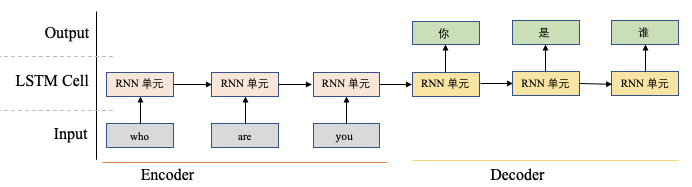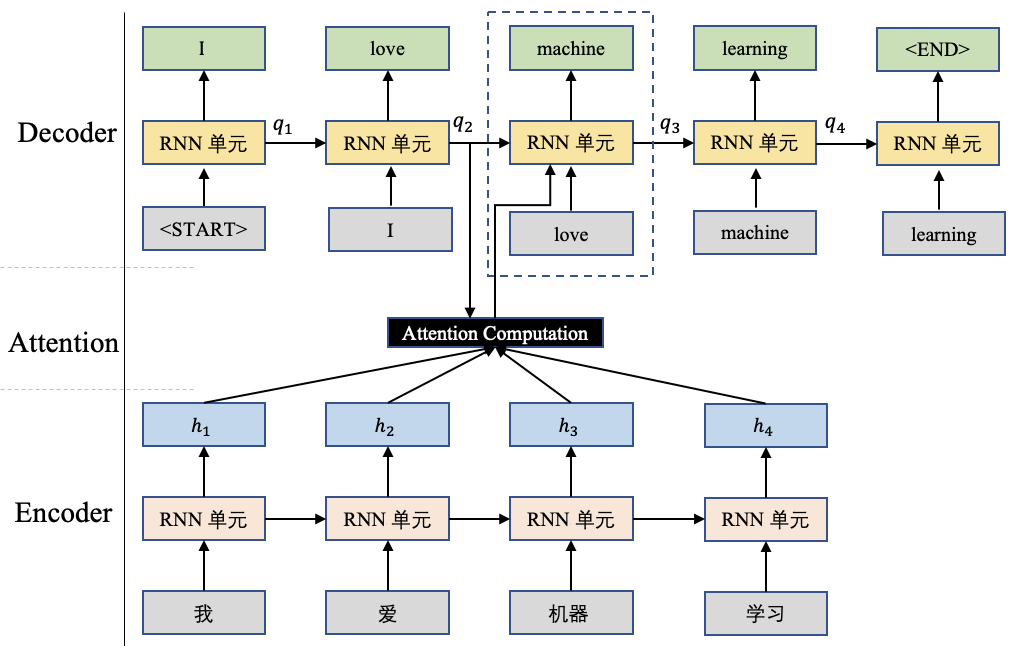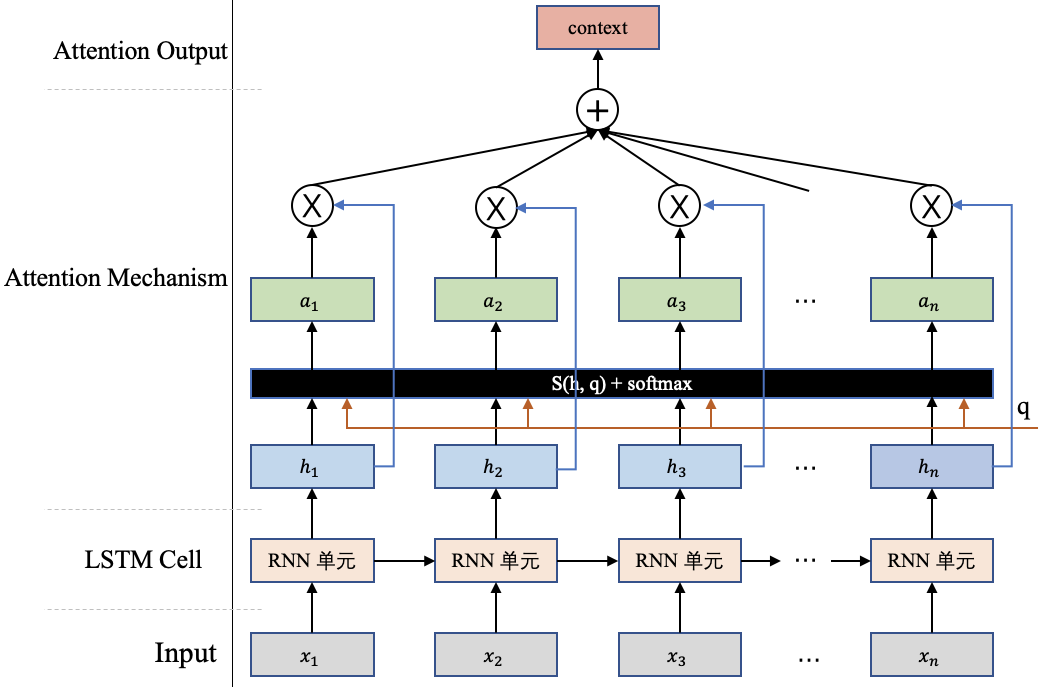## 1. 注意力机制是什么Attention机制自提出以来，出现了很多不同Attention应用方式，但大道是共同的，均是将模型的注意力聚焦在重要的事情上。本文后续将选择一些经典或常用的Attention机制展开讨论。

## 2. 经典注意力机制

### 2.1. 用机器翻译任务来看Attention机制的计算\begin{align} [a_1,a_2,a_3,a_4] &= softmax([s(q_2, h_1), s(q_2,h_2),s(q_2, h_3),s(q_2, h_4)]) \\ context&=\sum_{i=1}^4 a_i \cdot h_i \end{align}

### 2.2. 注意力机制的正式引入<center>图4 Attention机制图</center>

\begin{align}a_i = softmax(s(h_i, q))= \frac {exp(s(h_i,q))} {\sum_{j=1}^n exp(s(h_j, q))} \end{align}

$$context = \sum_{i=1}^n a_i \cdot h_i$$

• 加性模型: $s(h, q) = v^Ttanh(Wh+Uq)$
• 点积模型: $s(h, q) = h^Tq$
• 缩放点积模型: $s(h, q) =\frac{ h^Tq}{\sqrt D}$
• 双线性模型: $s(h, q) = h^TWq$

## 3. 注意力机制的一些变体

### 3.1. 硬性注意力机制

• 选择注意力分布中，分数最大的那一项对应的输入向量作为Attention机制的输出。
• 根据注意力分布进行随机采样，采样结果作为Attention机制的输出。

### 3.2. 键值对注意力机制

\begin{align} a_i=softmax(s(k_i,q)) = \frac{exp(s(k_i,q))}{\sum_{j=1}^n exp(s(k_j, q))} \end{align}

$$context=\sum_{i=1}^n a_i \cdot v_i$$

### 3.3. 多头注意力机制

\begin{align} a_{ij}=softmax(s(k_j,q_i)) &= \frac{exp(s(k_j,q_i))}{\sum_{t=1}^n exp(s(k_t, q_i))} \\ context_i &=\sum_{j=1}^n a_{ij} \cdot v_j \end{align}

$$context=context_1 \oplus context_2 \oplus context_3 \oplus...\oplus context_m$$

Last modification：September 18th, 2021 at 10:33 am